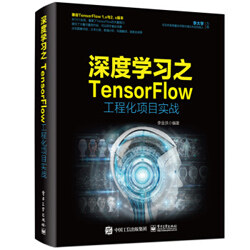# TensorFlow 工程实战（三）：结合知识图谱实现电影推荐系统## 实例描述

https://arxiv.org/pdf/1901.08907.pdf

### 一、准备数据集

https://github.com/hwwang55/MKR/tree/master/data/movie

• item_index2entity_id.txt：电影的 ID 与序号。具体内容如图 1 所示，第 1 列是电影 ID，第 2 列是序号。
• kg.txt：电影的知识图谱。图 2 中显示了知识图谱的 SPO 三元组（Subject-Predicate-Object），第 1 列是电影 ID，第 2 列是关系，第 3 列是目标实体。
• ratings.dat：用户的评分数据集。具体内容如图 3 所示，列与列之间用“::”符号进行分割，第 1 列是用户 ID，第 2 列是电影 ID，第 3 列是电影评分，第 4 列是评分时间（可以忽略）。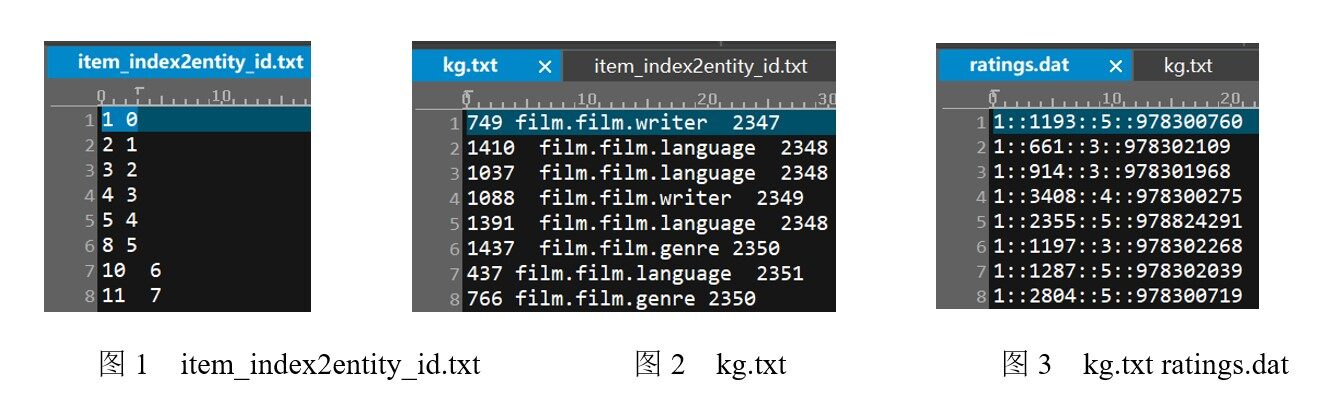### 二、预处理数据

• kg_final.txt：转化后的知识图谱文件。将文件 kg.txt 中的字符串类型数据转成序列索引类型数据，如图 4 所示。
• ratings_final.txt：转化后的用户评分数据集。第 1 列将 ratings.dat 中的用户 ID 变成序列索引。第 2 列没有变化。第 3 列将 ratings.dat 中的评分按照阈值 5 进行转化，如果评分大于等于 5，则标注为 1，表明用户对该电影感兴趣。否则标注为 0，表明用户对该电影不感兴趣。具体内容如图 5 所示。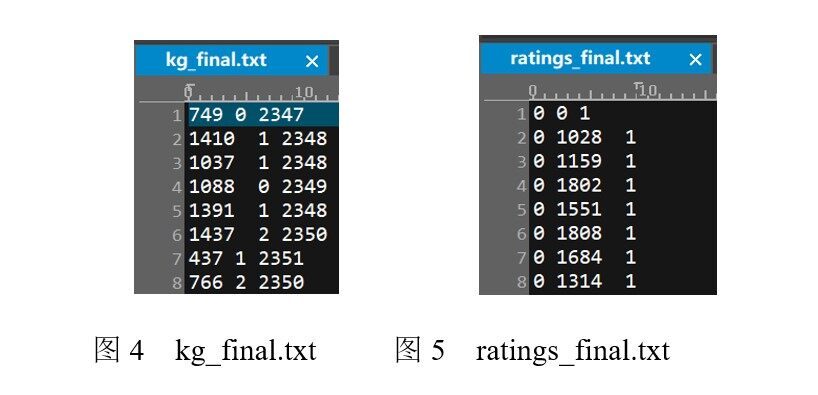### 三、搭建 MKR 模型

MKR 模型由 3 个子模型组成，完整结构如图 6 所示。具体描述如下。

• 推荐算法模型：如图 6 的左侧部分所示，将用户和电影作为输入，模型的预测结果为用户对该电影的喜好分数，数值为 0～1。
• 交叉压缩单元模型：如图 6 的中间部分，在低层将左右两个模型桥接起来。将电影评分数据集中的电影向量与知识图谱中的电影向量特征融合起来，再分别放回各自的模型中，进行监督训练。
• 知识图谱词嵌入（Knowledge Graph Embedding，KGE）模型：如图 6 的右侧部分，将知识图谱三元组中的前 2 个（电影 ID 和关系实体）作为输入，预测出第 3 个（目标实体）。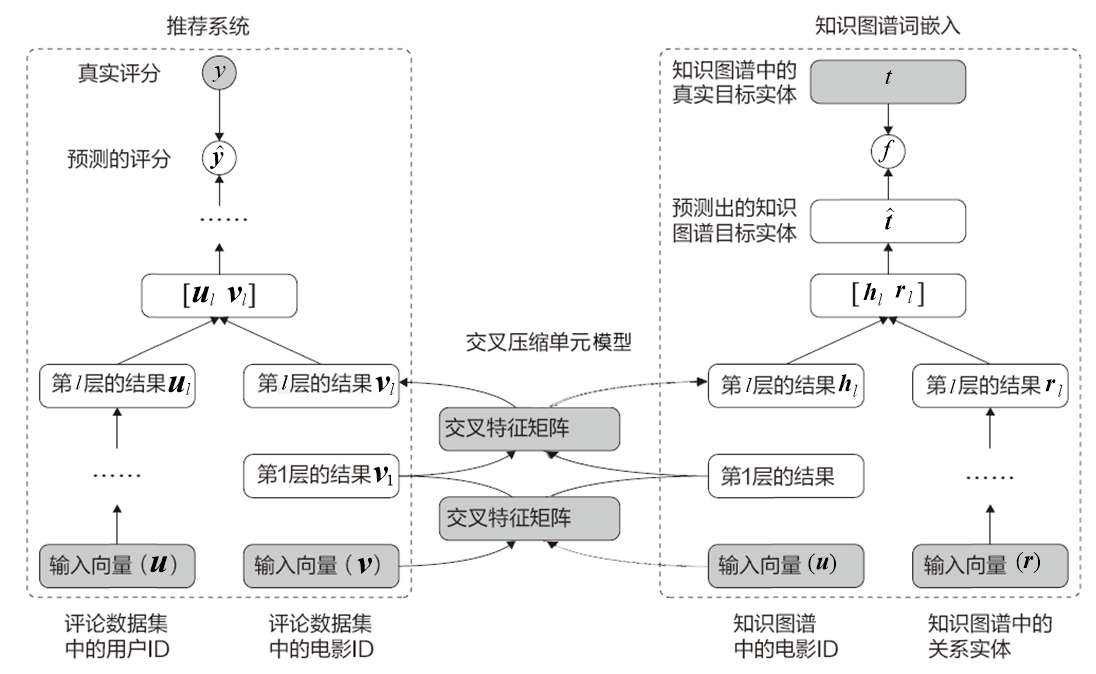#### 1. 交叉压缩单元模型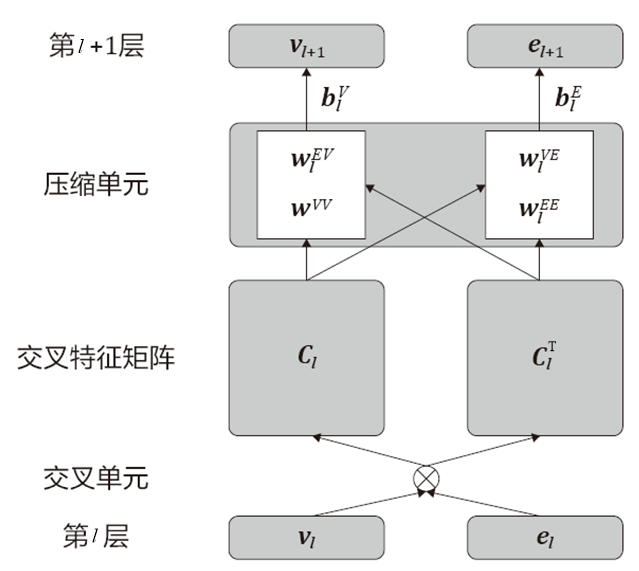（1）将 v_l 与 e_l 进行矩阵相乘得到 c_l。
（2）将 c_l 复制一份，并进行转置得到 c_l^T。实现特征交叉融合。
（3）将 c_l 经过权重矩阵 w_l^vv 进行线性变化（c_l 与 w_l^vv 矩阵相乘）。
（4）将 c_l^T 经过权重矩阵 w_l^ev 进行线性变化。
（5）将（3）与（4）的结果相加，再与偏置参数 b_l^v 相加，得到 v_(l+1)。v_(l+1) 将用于推荐算法模型的后续计算。
（6）按照第（3）、（4）、（5）步的做法，同理可以得到 e_(l+1)。e_(l+1) 将用于知识图谱词嵌入模型的后续计算。

import numpy as npimport tensorflow as tffrom sklearn.metrics import roc_auc_scorefrom tensorflow.python.layers import base class CrossCompressUnit(base.Layer):                  		#定义交叉压缩单元模型类    def __init__(self, dim, name=None):       super(CrossCompressUnit, self).__init__(name)       self.dim = dim       self.f_vv = tf.layers.Dense(1, use_bias = False)	#构建权重矩阵       self.f_ev = tf.layers.Dense(1, use_bias = False)       self.f_ve = tf.layers.Dense(1, use_bias = False)       self.f_ee = tf.layers.Dense(1, use_bias = False)       self.bias_v = self.add_weight(name='bias_v', 		#构建偏置权重                                           shape=dim,                                            initializer=tf.zeros_initializer())	       self.bias_e = self.add_weight(name='bias_e',                                            shape=dim,                                           initializer=tf.zeros_initializer())     def _call(self, inputs):        v, e = inputs					#v 和 e 的形状为 [batch_size, dim]        v = tf.expand_dims(v, dim=2)	#v 的形状为 [batch_size, dim, 1]        e = tf.expand_dims(e, dim=1)	#e 的形状为 [batch_size, 1, dim]         c_matrix = tf.matmul(v, e)#c_matrix 的形状为 [batch_size, dim, dim]        c_matrix_transpose = tf.transpose(c_matrix, perm=[0, 2, 1])        #c_matrix 的形状为 [batch_size * dim, dim]        c_matrix = tf.reshape(c_matrix, [-1, self.dim])        c_matrix_transpose = tf.reshape(c_matrix_transpose, [-1, self.dim])         #v_output 的形状为 [batch_size, dim]        v_output = tf.reshape(                           self.f_vv(c_matrix) + self.f_ev(c_matrix_transpose),                          [-1, self.dim]                                 ) + self.bias_v         e_output = tf.reshape(                          self.f_ve(c_matrix) + self.f_ee(c_matrix_transpose),                          [-1, self.dim]                                  ) + self.bias_e    #返回结果    return v_output, e_output

#### 2. 将交叉压缩单元模型集成到 MKR 框架中

• 低层：将所有的输入映射成词嵌入向量，将需要融合的向量（图 6 中的 v 和 h）输入交叉压缩单元，不需要融合的向量（图 6 中的 u 和 r）进行同步的全连接层处理。
• 高层：推荐算法模型和知识图谱词嵌入模型分别将低层的传上来的特征连接在一起，通过全连接层回归到各自的目标结果。

class MKR(object):    def __init__(self, args, n_users, n_items, n_entities, n_relations):        self._parse_args(n_users, n_items, n_entities, n_relations)        self._build_inputs()        self._build_low_layers(args) 	#构建低层模型        self._build_high_layers(args) 	#构建高层模型        self._build_loss(args)        self._build_train(args)     def _parse_args(self, n_users, n_items, n_entities, n_relations):        self.n_user = n_users        self.n_item = n_items        self.n_entity = n_entities        self.n_relation = n_relations         #收集训练参数，用于计算 l2 损失        self.vars_rs = []        self.vars_kge = []     def _build_inputs(self):        self.user_indices=tf.placeholder(tf.int32, [None], 'userInd')        self.item_indices=tf.placeholder(tf.int32, [None],'itemInd')        self.labels = tf.placeholder(tf.float32, [None], 'labels')        self.head_indices =tf.placeholder(tf.int32, [None],'headInd')        self.tail_indices =tf.placeholder(tf.int32, [None], 'tail_indices')        self.relation_indices=tf.placeholder(tf.int32, [None], 'relInd')    def _build_model(self, args):         self._build_low_layers(args)        self._build_high_layers(args)     def _build_low_layers(self, args):        #生成词嵌入向量        self.user_emb_matrix = tf.get_variable('user_emb_matrix',                                                       [self.n_user, args.dim])        self.item_emb_matrix = tf.get_variable('item_emb_matrix',                                                       [self.n_item, args.dim])        self.entity_emb_matrix = tf.get_variable('entity_emb_matrix',                                                       [self.n_entity, args.dim])        self.relation_emb_matrix = tf.get_variable('relation_emb_matrix',                                                      [self.n_relation, args.dim])         #获取指定输入对应的词嵌入向量，形状为 [batch_size, dim]        self.user_embeddings = tf.nn.embedding_lookup(                                      self.user_emb_matrix, self.user_indices)        self.item_embeddings = tf.nn.embedding_lookup(                                      self.item_emb_matrix, self.item_indices)        self.head_embeddings = tf.nn.embedding_lookup(                                     self.entity_emb_matrix, self.head_indices)        self.relation_embeddings = tf.nn.embedding_lookup(                              self.relation_emb_matrix, self.relation_indices)        self.tail_embeddings = tf.nn.embedding_lookup(                                   self.entity_emb_matrix, self.tail_indices)         for _ in range(args.L):#按指定参数构建多层 MKR 结构            #定义全连接层            user_mlp = tf.layers.Dense(args.dim, activation=tf.nn.relu)            tail_mlp = tf.layers.Dense(args.dim, activation=tf.nn.relu)            cc_unit = CrossCompressUnit(args.dim)#定义 CrossCompress 单元            #实现 MKR 结构的正向处理            self.user_embeddings = user_mlp(self.user_embeddings)            self.tail_embeddings = tail_mlp(self.tail_embeddings)            self.item_embeddings, self.head_embeddings = cc_unit(                               [self.item_embeddings, self.head_embeddings])            #收集训练参数            self.vars_rs.extend(user_mlp.variables)            self.vars_kge.extend(tail_mlp.variables)             self.vars_rs.extend(cc_unit.variables)            self.vars_kge.extend(cc_unit.variables)                            def _build_high_layers(self, args):        #推荐算法模型        use_inner_product = True 		#指定相似度分数计算的方式        if use_inner_product:    		#内积方式            #self.scores 的形状为 [batch_size]            self.scores = tf.reduce_sum(self.user_embeddings * self.item_embeddings, axis=1)        else:            #self.user_item_concat 的形状为 [batch_size, dim * 2]            self.user_item_concat = tf.concat(                       [self.user_embeddings, self.item_embeddings], axis=1)            for _ in range(args.H - 1):                rs_mlp = tf.layers.Dense(args.dim * 2, activation=tf.nn.relu)                #self.user_item_concat 的形状为 [batch_size, dim * 2]                self.user_item_concat = rs_mlp(self.user_item_concat)                self.vars_rs.extend(rs_mlp.variables)            #定义全连接层            rs_pred_mlp = tf.layers.Dense(1, activation=tf.nn.relu)            #self.scores 的形状为 [batch_size]            self.scores = tf.squeeze(rs_pred_mlp(self.user_item_concat))            self.vars_rs.extend(rs_pred_mlp.variables)  #收集参数        self.scores_normalized = tf.nn.sigmoid(self.scores)         #知识图谱词嵌入模型        self.head_relation_concat = tf.concat(  #形状为 [batch_size, dim * 2]                  [self.head_embeddings, self.relation_embeddings], axis=1)        for _ in range(args.H - 1):            kge_mlp = tf.layers.Dense(args.dim * 2, activation=tf.nn.relu)            #self.head_relation_concat 的形状为 [batch_size, dim* 2]            self.head_relation_concat = kge_mlp(self.head_relation_concat)            self.vars_kge.extend(kge_mlp.variables)        kge_pred_mlp = tf.layers.Dense(args.dim, activation=tf.nn.relu)        #self.tail_pred 的形状为 [batch_size, args.dim]        self.tail_pred = kge_pred_mlp(self.head_relation_concat)        self.vars_kge.extend(kge_pred_mlp.variables)        self.tail_pred = tf.nn.sigmoid(self.tail_pred)         self.scores_kge = tf.nn.sigmoid(tf.reduce_sum(self.tail_embeddings * self.tail_pred, axis=1))        self.rmse = tf.reduce_mean(            tf.sqrt(tf.reduce_sum(tf.square(self.tail_embeddings - self.tail_pred), axis=1) / args.dim))

• 使用内积的方式，计算用户向量和电影向量的相似度。有关相似度的更多知识，可以参考 8.1.10 小节的注意力机制。
• 将用户向量和电影向量连接起来，再通过全连接层处理计算出用户对电影的喜好分值。

（1）将电影向量和知识图谱中的关系向量连接起来。

（2）将第（1）步的结果通过全连接层处理，得到知识图谱三元组中的目标实体向量。

（3）将生成的目标实体向量与真实的目标实体向量矩阵相乘，得到相似度分值。

（4）对第（3）步的结果进行激活函数 sigmoid 计算，将值域映射到 0～1 中。

#### 3. 实现 MKR 框架的反向结构

MKR 框架的反向结构主要是 loss 值的计算，其 loss 值一共分为 3 部分：推荐算法模型模型的 loss 值、知识图谱词嵌入模型的 loss 值和参数权重的正则项。具体实现的代码如下。

    def _build_loss(self, args):        #计算推荐算法模型的 loss 值        self.base_loss_rs = tf.reduce_mean(            tf.nn.sigmoid_cross_entropy_with_logits(labels=self.labels, logits=self.scores))        self.l2_loss_rs = tf.nn.l2_loss(self.user_embeddings) + tf.nn.l2_loss (self.item_embeddings)        for var in self.vars_rs:            self.l2_loss_rs += tf.nn.l2_loss(var)        self.loss_rs = self.base_loss_rs + self.l2_loss_rs * args.l2_weight         #计算知识图谱词嵌入模型的 loss 值        self.base_loss_kge = -self.scores_kge        self.l2_loss_kge = tf.nn.l2_loss(self.head_embeddings) + tf.nn.l2_loss (self.tail_embeddings)        for var in self.vars_kge: 	#计算 L2 正则            self.l2_loss_kge += tf.nn.l2_loss(var)        self.loss_kge = self.base_loss_kge + self.l2_loss_kge * args.l2_weight     def _build_train(self, args): 	#定义优化器        self.optimizer_rs = tf.train.AdamOptimizer(args.lr_rs).minimize(self.loss_rs)        self.optimizer_kge = tf.train.AdamOptimizer(args.lr_kge). minimize(self. loss_kge)     def train_rs(self, sess, feed_dict):		#训练推荐算法模型        return sess.run([self.optimizer_rs, self.loss_rs], feed_dict)     def train_kge(self, sess, feed_dict): 		#训练知识图谱词嵌入模型        return sess.run([self.optimizer_kge, self.rmse], feed_dict)     def eval(self, sess, feed_dict):			#评估模型        labels, scores = sess.run([self.labels, self.scores_normalized], feed_dict)        auc = roc_auc_score(y_true=labels, y_score=scores)        predictions = [1 if i >= 0.5 else 0 for i in scores]        acc = np.mean(np.equal(predictions, labels))        return auc, acc     def get_scores(self, sess, feed_dict):        return sess.run([self.item_indices, self.scores_normalized], feed_dict)

### 四、训练模型并输出结果

……
epoch 9 train auc: 0.9540 acc: 0.8817 eval auc: 0.9158 acc: 0.8407 test auc: 0.9155 acc: 0.8399